MPA-HOMEPAGE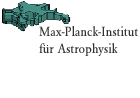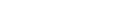Quickfinder FAQ job vacancies   about the institute research groups local phone list weekly seminars preprints library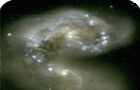Volume Simulations: Cluster Catalogs from Light Cone DatasetsMPA Homepage > Scientific Research > Research Groups Cluster CatalogGo to: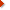Data Visualization (images & movies from Numerical Simulation projects)VIRGO MembersVIRGO Links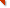Main U.K. SitePublic Announcement
of the VIRGO data
releaseLight-cone Surveys

Five separate light-cone 'sky' surveys are produced by each run. Their properties are summarized in the following table -

Lightcone geometry : LCDM

 Survey type Angular coverage R_max(Mpc/h) z_max octants pi/2ster 3000 1.46 oct+wedge(*) pi/2 ster 6000 6.8 spheres 4pi ster 1500 0.58 deep wedge 100 sqdeg 5100 4.37

Lightcone geometry : tCDM

 Survey type Angular coverage R_max(Mpc/h) z_max octants pi/2ster 2000 1.25 spheres 4pi ster 1000 0.44 deep wedge 100 sqdeg 3400 4.60

* available for LCDM only. The POW survey is a combination of the PO octant (extending to z=1.46) plus a 16x76 sq deg wedge extending to twice the simulation cube length (6000/h Mpc) using periodic boundaries. The center of the wedge is co-aligned with the x-y diagonal plane in the simulation cube.The surveys are centered at the following locations in the original simulation cube (mapped to 0-1 interval) -larger version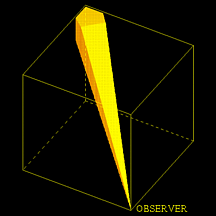DW has origin (0,0,0) and is directed toward (1,1,1)larger version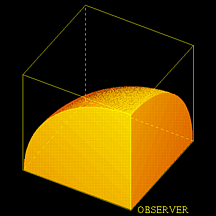PO has origin (0,0,0) and is directed toward (1,1,1)larger version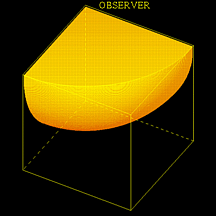NO has origin (1,1,1) and is directed toward (0,0,0)larger version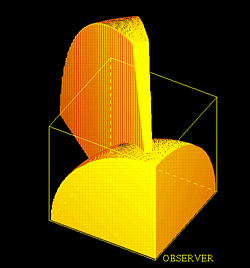POW has origin (0,0,0) and is directed toward (1,1,larger version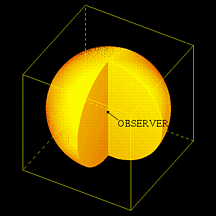MS has origin (0.5,0.5,0.5)

VS has origin (0,0,0) and uses octants from that location plus the 7 other vertices (periodic replications of the origin)Cluster Catalog Files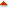Cluster catalogs derived from a SPHERICAL OVERDENSITY method applied to the light-cone particle data. Cluster lists are found in files of the form

grpSO.d$delta_c/$model.$survey.msort$min

where

$delta_c = { 200, 500 } is the defining density threshold$model = { tcdm, lcdm } is the cosmological model
$survey = { DW, VS, MS, NO, PO/POW } is the choice of sky survey$min = { 12, 48 } is the minimum particle count of the cluster

Each file contains cluster information

#     m     zred     sigma     ip     x     y     z     vx     vy     vz

where

m = number of particles (particle mass is 2.22/2.25e12/h for tcdm/lcdm)
zred = redshift
sigma = measured 1D velocity dispersion
ip = parent flag (see below)
x,y,z = cluster location in SURVEY COORDINATES in 0-1 units
vx,vy,vz= physical peculiar velocity in km/s

In the cluster data sets, survey coordinate systems are defined so that the survey origins are located at

PO/NO/DW : (0,0,0)
VS/MS : (0.5,0.5,0.5)

Cluster locations in the ORIGINAL cube volume can be recovered by

NO : x -> 1-x
VS : x -> mod( (x-0,5), 1 )
PO/DW/MS : no transformation requiredCluster Finding AlgorithmClusters are identified using a spherical overdensity method with the following steps:

1. measure distance to 8th nearest particle = r8
2. sort in r8 (increasing order) & identify first particle
3. center group on this particle
2. cut particle list at overdensity threshold \delta_c (1)
3. flag particles as belonging to this group (2)
4. find next particle in r8 list not flagged as a group member
5. repeat steps 3 & 4 until max r8 is reached (3)

Notes:

1. \delta_c is RELATIVE TO CRITICAL DENSITY \rho_c(z) for both LCDM and tCDM
2. particles can belong to more than one group
3. defined by comparing density constrast using r8 to \delta_c threshold

### LIMITATIONS ###
No attempt is made to clean near boundaries of the datasets. Some incompleteness near the planar and radial edges of the surveys is present. If volume completeness is desired, stay interior to ~3/h Mpc of any boundary.

+ IPARENT flag
The issue of ovelapping clusters is handled as follows. A cluster is defined to be a parent if it is the largest of any group of clusters with which it overlaps. Isolated clusters are parents by default. The `family' criterion employed is that the threshold spheres of pair i & j overlap:

| cen_i - cen_j | <= Rvir_i + Rvir_j

Note that the center of any cluster cannot lie within the threshold radius of any cluster other than itself.Additonal Cluster DataThe catalog files are shortened versions of the original group finder output. The group finder processes planes of cubes in the Z-direction. Separate output files for each Z-slice are produced. These files can be made available upon request.

For each slice iz, there are three output files:

• z$iz.base : basic cluster data • z$iz.extra : internal cluster structure data
• z\$iz.iparent : list of binary values for iparent flag (see above)

The *.base files contain:

#   M    {X Y Z}_c    {Vx Vy Vz V}_com    sigma1D    R    R.5    S.5    {X Y Z}_mb
{X Y Z}_com

where

 M {X Y Z}_c {Vx Vy Vz V}_com sigma1D R R.5 S.5 {X Y Z}_mb {X Y Z}_com = cluster mass in particles (1 particle = 2.2e12/h Msol in both models) = cluster center (1) = center-of-mass velocity components and speed (2) = one-dimensional velocity dispersion (2) = cluster radius (1) = ratio of half mass radius to full radius R_{1/2}/R = ratio of velocity dispersions within R_{1/2} and R = position of most bound cluster member (1,3) = position of cluster center-of-masss (1)

Notes:

1. in box units (L=1). Box is (2/3)000/h Mpc for (t/L)cdm models.
2. in km/s
3. determined using N^2 sum of potential among group members only.

The *.extra files contain moments of the internal density and velocity:

#   {Rxx Rxy Rxz Ryy Ryz Rzz}   {Vxx Vxy Vxz Vyy Vyz Vzz}

where

Rij = Sum_k (R-R_c)_i (R-R_c)_j / M
Vij = Sum_k (V-V_com)_i (V-V_com)_j / M
and the sum is over members k running from 1 to M.

The *.iparent files contain the integer iparent flag for each cluster.

Original text file written by August.E.Evrard.Comments to: Virgo Administrator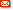virgo@mpa-garching.mpg.de